## Pneumatics: Force and Pressure

1. Give examples of forces and when they are needed?
2. Identify direction and magnitude of different forces
3. Describe the relationship between mass and weight and the units kilogram and Newton?
4. Describe the attractive force between the moon and earth?
5. What is leverage and when is it used?
6. What is a dynamometer?
7. What is the center of gravity?
8. Who was Isaac Newton?
9. Describe the relationship between pressure, force and area? What units are there for pressure?
10. Why use snowshoes?
11. Describe atmospheric pressure, overpressure, underpressure and vacuum. Give examples?
12. What is a Barometer?
13. What happens to a trapped gas if its temperature is increased?
14. Questions

## Force

Give examples of forces and when they are needed?

Gravitational force:
Gravity affects all matter (things that are and have mass) on earth. This keeps us humans left on earth and we do not float away. Just as an object is pulled towards the earth the earth is pulled towards the object.

Other forces:
Muscles, engines and magnets can also create forces. The friction force is the force that resists when you pull e.g. a box on the ground.

Identify direction and magnitude of different forces

Force is given with arrows. The greater the force, the longer the arrow. You can also print out the amount of force. Power is given in Newton (N). A force is always held back by a counteracting force.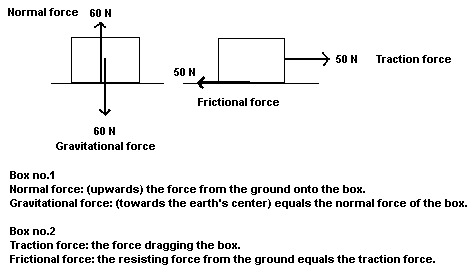A force can be directed in other directions also. Here are two forces replaced with a force of 70 N. The dashed lines are drawn to help when you draw the replacement force.Describe the relationship between mass and weight and the units kilogram and Newton?

The mass describes how much matter an object has. Mass has the unit kilograms (kg). The weight of the mass is the attractive force towards the earth’s center. A certain mass thus has a certain attractive force towards the earth’s center.G = gravity, attractive force, weight, gravitational force (unit specified in Newtons (N))
m = mass (kg)
g = gravity coefficient (m/s2) = 10 m/s2 on Earth (Jupiter has approx. 25 m/s2)

Calculate with the formula above, how much weight your mass has on earth.

Describe the attractive force between the moon and earth?

All masses are attracting each other. Earth affects the moon with a certain attractive force. The moon affects earth with the same attractive force. It is always a force holding back; otherwise the moon would be slung into space. This type of attractive force is called gravity.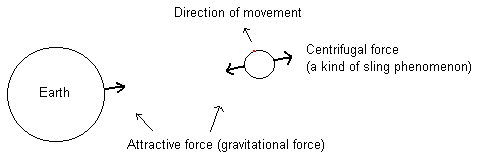What is leverage and when is it used?With the help of a lever you can move large stones. A gearbox in a car is also based on leverage. Small gearwheels pull bigger gearwheels, which gives greater force.

What is a dynamometer?

A dynamometer measures forces.The greater the force pulling the hook, the greater the force shows on the graduated scale in the tube. Get a dynamometer and test what forces that may arise. A dynamometer is often also graduated in grams (mass).

What is the center of gravity?

An object's center of gravity is the point where we can say all its mass is concentrated. An iron ball has its center of gravity in the middle. When balancing a pen on your finger the center of gravity is just above your finger, centered in the middle of the pen. Below are shown center of gravity points in two figures. Note that although figure 1 is a cylinder, its center of gravity is in the middle of the volume.Figure 1                               Figure 2

If you tilt a geometric figure the attractive force (gravitational force) from the center of gravity wanders outside the object’s supporting surface. When this happens the object overturns by itself. Place a milk carton on a table and start tilting it until it is overturns by itself. When this happens the center of gravity is outside the supporting surface.

Who was Isaac Newton?Isaac Newton lived in England from 1643 to 1727. He studied the gravitational force and named its unit (Newton). It is said that Newton began investigating the laws of gravity when seeing an apple falling from a tree.

## Pressure

Describe the relationship between pressure, force and area? What units are there for pressure?

Formula: P = F / A
P = Pressure (unit: Pa or N/m2)
F = Force (N)
A = Area (m2)

The unit of pressure is Pa (Pascal). Pressure is also measured in "bar", "atmospheric pressure" and "millimeters of mercury”. Pressure means that a certain force works on a given area.

Why use snowshoes?

A person's weight is held up by an opposing equal force working from below and upwards on the sole of the feet. All weight (force) is distributed on the feet sole areas. If the area is larger, it means that the pressure against the surface becomes smaller. In this way a man with snowshoes does not sink so deep in the snow. Look at the formula above. Insert a larger area in the formula and see what happens with the pressure.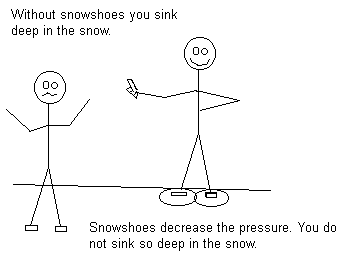Describe atmospheric pressure, overpressure, underpressure and vacuum. Give examples?

Air pressure:
The air (including nitrogen, oxygen, etc.) that exists above the earth’s surface has a certain mass. The mass has a certain gravitational force (weight) on earth. The air total weight on one square meter on Earth's surface generates a pressure here. This pressure is called one atmospheric pressure or one bar. An atmospheric pressure weighs as much as a ten-meter high column of water. Which is the amount of one atmospheric pressure expressed in Pa?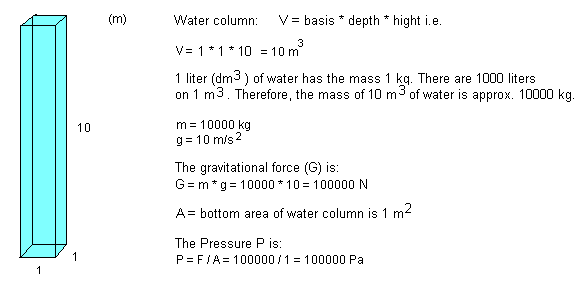One atmospheric pressure or one bar is according to the above calculation 100 000 Pa.

Overpressure
If you pump air into a closed tin the air pressure increases in the tin. The pressure here is higher than outside the tin. It is an overpressure in the tin.

Underpressure
If air instead is sucked out of a closed tin, the pressure inside the tin is lower than outside the tin. It is an underpressure in the tin.

Vacuum
If air is sucked out of the tin until no air remains, it is a vacuum in the tin. In space there in no air at all. This means we have a constant vacuum in space.

The Magdeburg hemispheres

Otto van Guericke (1654) showed a famous experiment. He made two hemispheres of metal that just fit into each other. Otto invented an air pump that sucks out air from the created sphere. Eight horses (different data of how many) were connected to one hemisphere and eight horses to the other. The horses tried to pull apart the sphere. The negative pressure (underpressure) existing in the sphere was so big that the sphere could not be divided despite all horsepowers. The reason was that the air pressure outside the sphere was bigger than the inside pressure. The air outside the sphere squeezed the hemispheres (ball halves) together with such force that they could not be divided. This way it was shown that the air pressure we humans are staying in is quite big.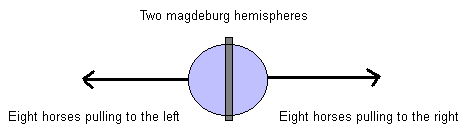What is a Barometer?

A barometer measures air pressure.What happens to a trapped gas if its temperature is increased?

If instead a balloon is heated, the balloon will grow is size, since the air pressure inside the balloon would be bigger. Keep in mind that it is always a counterpressure holding back as the balloon gets bigger.

Questions

1. Calculate the weight (attractive force) of your mass on earth?
2. Place a milk carton on a table and start tilting it until it overturns by itself. Do the same when the milk carton is lying down. How can you do to get its center of gravity?
3. Get a dynamometer and test what forces can occur?
4. Give examples of where we have positive pressure (overpressure) and negative pressure (underpressure)?

• NGU Science
• JAN 1, 2012
• FEB 4, 2012
• Admin
##### The course is compiled by Admin

These basic courses are compiled by Lars Helge Swahn. They are designed for 15-16 year old students in order to reach basic skills in science. The courses do also provide an easier way to understand the NGU (AIC) gnostic teachings.

#### Extra information

Copywrite NGU, Northern Pontifical Academy 2019 (A.I.C.)